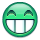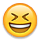#### Howdy, Stranger!

It looks like you're new here. If you want to get involved, click one of these buttons!

# How do I Find a non numeric value in a string?

edited 2006-07-07
I'm trying to find the position of the first value that is not a numeric value within a string.

pTxtNumber is passed in and could be 10-15672 for example. So I want to return position 3 because this is the first non numeric character. Then I want to chop of the string up to and including this character so that I am left with 15672.

This code i initially did checks for where the numeric value begins but now i want to do the opposit.

FOR i := 1 TO STRLEN(pTxtNumber) DO BEGIN
IF Pos = 0 THEN
IF FORMAT(pTxtNumber) IN THEN
Pos := i;
END;

Any help would be greatly appreciated.

Cheers

Mike

• In place of

IF FORMAT(pTxtNumber) IN THEN

put

IF NOT (FORMAT(pTxtNumber) IN ) THEN

I think it could work ...
• 'The prefix operator cannot be used on text'. I also tried:

IF FORMAT(pTxtNumber) NOT IN THEN

and this did not work either.

I suppose i could enter into a substring 'abcdefg-!"£\$%^&*' etc and then check in my string to see if it contains any of the characters in the substring but i thought there might be a more efficient way of saying find the first non-numeric character.

Any ideas?
• Posts: 8,737Member, Moderator
``````txtString := '10-15672';
txtNoNumbers := DELCHR(txtString,'=','1234567890'); // remain only non-number chars and the first char in the resulting string will be the first non-numeric char in the original string
WHILE txtNoNumber <> '' DO BEGIN
// take the first char of "txtNoNumber" (there can be multiple non-numeric chars)
// and give me the position where it is in "txtString"
intNoNumber := STRPOS(txtString,COPYSTR(txtNoNumber,1,1));

// "txtString" now starts AFTER the first non-numeric char
txtString := COPYSTR(txtString,intNoNumber + 1);

// lets check if there are other non-numeric chars
txtNoNumbers := DELCHR(txtString,'=','1234567890');
END;

MESSAGE('The string "txtString" (=%1) doesn''t contain non-numeric chars',txtString);
``````
Regards,Alain Krikilion
Use the SEARCH,Luke! || No PM,please use the forum. || May the <SOLVED>-attribute be in your title!
NAV TechDays 2019: 21 & 22 November 2019, Antwerp (Belgium)
• If the numbers are always separated by a dash then you could do:
``````TargetString := DELCHR(SourceString,'=','-');
``````
if there are more saparation characters, (like . or ,) you could do:
``````TargetString := DELCHR(SourceString,'=','-.,');
``````
if you want to remove letters you do:
``````TargetString := DELCHR(SourceString,'=','abcdefghijklmnopqrstuvwxyz');
``````
etcetera

<edit>so that still doesn't give you the position of the first non numeric character..... let me think about that</edit>
• SourceString - Text30
TargetString - Text30
IFoundIt - boolean
MyPos - Integer
``````MyPos := 0;
IFoundIt := FALSE;
SourceString := '123-456';
REPEAT
MyPos += 1;
IF DELCHR(COPYSTR(SourceString,MyPos,1),'=','0123456789') <> '' THEN
IFoundIt := TRUE;
IF MyPos = STRLEN(SourceString) THEN BEGIN
IFoundIt := TRUE;
MyPos := 0; // no non-numeric value found, so return 0
END;
UNTIL IFoundIt;
MESSAGE('position is ' + format(MyPos));
``````
That should find the first non-numeric character in SourceString. So then you wrap it in a function and use return value.
``````
mystr := '10-98765';

FOR i := 1 TO STRLEN(mystr) DO BEGIN
IF NOT (mystr[i] IN [48..57]) THEN BEGIN  // ascii for 0 thru 9
newstr := COPYSTR(mystr,i+1);
i := STRLEN(mystr);  // drops out of for statement
END;

END;

MESSAGE('%1',newstr)
``````
• so how do you get the ascii value out of the char? I tried with MESSAGE, but that returns the alphanumeric character.
• Chars can be treated as integers

AsciiNo is integer
mystr is text
``````
mystr := '10-2376'
AsciiNo:= mystr;  // save char value as integer (ie the ascii value)

message('ASCII No. is %1',AsciiNo);

``````

Message returns 48 which is ASCII No. for 0 (zero)
• ``````  i := 1;
while (mystr[i] in ['0'..'9']) and (i<= StrLen(mystr)) do
i := i+1;

if i>StrLen(mystr) then
Exit(0)    //only numbers in string...
else
Exit(i);    //return the position of the non-numeric character
``````

That's all folks... 8)
Kamil Sacek
MVP - Dynamics NAV
My BLOG
NAVERTICA a.s.
• Yeah right, with code samples from two other people I can write really efficient code too• I just added the = FALSE to the second line. This then tells me the line number where the first non-numeric character is.

FOR i := 1 TO STRLEN(pTxtNumber) DO BEGIN
IF FORMAT(pTxtNumber) IN = FALSE THEN
Pos := i;
END;

IntNumber := 0;
IF Pos <> 0 THEN BEGIN
Alpha := DELSTR(pTxtNumber, 1, Pos);
EVALUATE(IntNumber, Alpha);
END;
EXIT(IntNumber);

Thanks for the help!!!
Mike
• If your goal is to generate an error if the code contains a non numeric character, why not just do EVALUATE(MyInt,TheWholeThing)?
• The code below by Spost29 does just the trick. Mine works but this is a lot clearer.

I just wanted to take the part of the string after the non-numeric character which in this case is '-'. So i wanted to return 98765.

mystr := '10-98765';

FOR i := 1 TO STRLEN(mystr) DO BEGIN
IF NOT (mystr IN [48..57]) THEN BEGIN // ascii for 0 thru 9
newstr := COPYSTR(mystr,i+1);
i := STRLEN(mystr); // drops out of for statement
END;

END;

MESSAGE('%1',newstr)
``````mystr := '10-98765';
• Thanks for that. Will give it a try on Monday!!!• the gift that keeps givingI think it is so cool that these old posts are still helping people